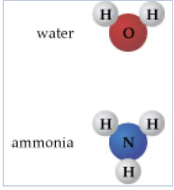Problem: Part A. What is the ratio of hydrogen atoms (H) to oxygen atoms (O) in 2 L of water? Enter the simplest whole number ratio in order of hydrogen to oxygen, respectively. Express your answer as two integers, separated by a comma (e.g., 3,4).Using mass measurements, early chemists were able to determine that for pure substances, the mass proportions of the composite elements were the same, no matter how much of the substance was analyzed. This became known as the law of definite proportions, or the law of constant composition. Dalton interpreted that this constant mass composition arose from constant composition of atoms. Working with this concept will help you to better understand the particulate nature of matter. To answer the questions, refer to the structures of water and ammonia shown here.

FREE Expert Solution
88% (318 ratings)Problem Details

Part A. What is the ratio of hydrogen atoms (H) to oxygen atoms (O) in 2 L of water?

Enter the simplest whole number ratio in order of hydrogen to oxygen, respectively. Express your answer as two integers, separated by a comma (e.g., 3,4).

Using mass measurements, early chemists were able to determine that for pure substances, the mass proportions of the composite elements were the same, no matter how much of the substance was analyzed. This became known as the law of definite proportions, or the law of constant composition. Dalton interpreted that this constant mass composition arose from constant composition of atoms. Working with this concept will help you to better understand the particulate nature of matter. To answer the questions, refer to the structures of water and ammonia shown here.